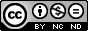### Basic Engineering Drawing - Preliminary Geometry

Introduction
1.         Engineering drawing is the application of the principles and methods of geometry.  Without the knowledge of geometry it is difficult to prepare a drawing. All drawings have points, lines and angles. Geometrical drawing is the foundation of all engineering drawing. Accuracy, neatness and legibility are of great importance in engineering drawing.

2.         Geometrical drawing is the art of representation of geometrical objects such as square, rectangle, cube, cylinder, cone, sphere, etc. Geometrical drawing consists of:
(a)       Plane Geometrical Drawing.        It is the representation of objects on a drawing sheet, having two dimensions, i.e. length, breadth and radius such as square, rectangle, parallelogram, circle, etc.
(b)       Solid Geometrical Drawing.         It is the representation of objects on a drawing sheet, having three dimensions, i.e. length, breadth and height such as cube, cylinder, cone, sphere, etc.
Terms
3.         Point.             According to Euclid, it is something that has position but no dimensions.  A point is marked by a dot in engineering drawing and denoted by capital letters A, B, C…
4.         Lines.             Lines are of various types as given below:
(a)       Straight Line.           lt is the shortest distance between two points joined by a series of points overlapping each other.
(b)       Curved Line.            A curved line is a line which continuously changes direction. It is neither broken nor a straight line.
(c)        Horizontal Line.      In a three dimensional object, a horizontal line is a line which is parallel to the surface of earth looked upon as a plane. In engineering drawing, a line drawn parallel to base line is known as a horizontal line.
(d)       Vertical Line.            It is a straight line perpendicular to the horizontal line.
(e)       Oblique Line.           It is a straight line, which is neither vertical nor horizontal.
(f)        Parallel Lines.          Two lines are said to be parallel if these lie in the same plane and do not meet however far they are extended.
5.         Angle.            Two straight  lines meeting at a point make an angle. An angle is the included inclination of the two lines. [Refer Fig 1(a) and (b)]
(a)       Acute Anqle.             It is an angle numerically smaller than a right angle.
(a)          Obtuse Angle.         An obtuse angle is an angle greater than a right angle and smaller than a straight angle.
(b)          Straight Angle.        It is an angle whose sides lie on the same straight line, but extend in opposite directions from the vertex. It is an angle of 180°.
(c)          Reflex Angle.           It is an angle, which is greater than 180O and less than 360O.

Fig. 1(a)  Various Angles
(d)          Adjacent Angles.    Two angles having a common side, a common vertex and lying on opposite sides of their common side are termed as adjacent angles.
(e)          Complementary Angles.  Two angles whose sum is a right angle are termed as complementary angles. The two acute angles in a right triangle are always complementary to each other.
(f)           Supplementary Angles.    Two angles whose sum is 1800 are termed as supplementary angles.
Fig. 1(b)  Various Angles

6.         Chord.           It is a straight line joining any two points on the circumference of a circle.  The longest chord of a circle is its diameter.
7.         Tangent to a Circle.           It is a straight line that passes through exactly one point on the circumference of the circle. A tangent is always at right angle to the radius passing through the point of contact.
Fig. 1(c)  Chord and Tangent